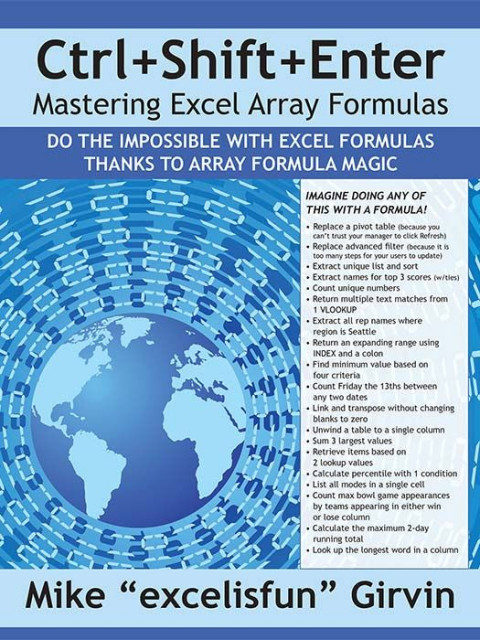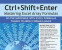### Ctrl+Shift+Enter Array Formula PDF e-Book

Master Excel Array Formulas

The ultimate guide to doing the impossible in Excel with array formulas. Starts from the basics, through formulas to extract unique lists to a new range.

Ctrl+Shift+Enter PDF e-Book
A Book About Building Efficient Formulas, Advanced Formulas, and Array Formulas for Data Analysis and Calculating Problems by Mike "ExcelisFun" Girvin
Designed with Excel gurus in mind, this handbook outlines how to create formulas that can be used to solve everyday problems with a series of data values that standard Excel formulas cannot or would be too arduous to attempt. Beginning with an introduction to array formulas, this manual examines topics such as how they differ from ordinary formulas, the benefits and drawbacks of their use, functions that can and cannot handle array calculations, and array constants and functions. Among the practical applications surveyed include how to extract data from tables and unique lists, how to get results that match any criteria, and how to utilize various methods for unique counts. This book contains 529 screen shots.

#### Affiliates

Like this product?

Spread the word about it and earn 20.00% of the purchase price on sales you refer.